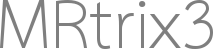# Question about 'design matrix' and results of 'fwe_pvalue' when using connectomestats nbse

Hi all MRtrix3 experts,
I have got the connectivity matrix of two group, one control group and one patient group. Every group has six subjects. Now I want to use connectomestats (nbse algorithm) to analyze changes of connectivity between any two nodes. Here I have total 32 nodes, 16 on the left hemisphere and 16 on the right hemisphere.
I have two questions.

(1) I am not sure if the design matrix and contrast vector I wrote is correct. And would you like to check them for me.

design matrix & contrast vector of mine

design matrix
1 1 0
1 1 0
1 1 0
1 1 0
1 1 0
1 1 0
1 0 1
1 0 1
1 0 1
1 0 1
1 0 1
1 0 1
the first six rows represent control group, and the last six rows represent patient group
*** contrast vector
0 1 -1

(2) when the element whose value is lower than 0.05 in “fwe_pvalue.csv” , do it mean the connectivity has significant changes between respective two nodes of two group ?
and what is it mean when the value is zero?

Thanks very much for your patience.

Zhaoqing

1 Like

(1) I am not sure if the design matrix and contrast vector I wrote is correct. And would you like to check them for me.

Your design matrix is not ideal, as it is rank-deficient: the first column can be represented as the sum of the second and third columns, which means that there are an infinite number of solutions to the model fit. You want to either have one column per group, and extract the difference between them:

``````1  0
1  0
0  1
0  1

1 -1
``````

, or capture the population mean in one column and the group difference in another:

``````1  1
1  1
1 -1
1 -1

0  1
``````

(2) when the element whose value is lower than 0.05 in “fwe_pvalue.csv” , do it mean the connectivity has significant changes between respective two nodes of two group? and what is it mean when the value is zero?

Beware that the contents of file “`fwe_pvalue.csv`” in fact contains the values (1-p), where p is the familywise error corrected p-value. So a value of ~1 in that file indicates a p-value of ~0, which would mean that the effect observed in that edge exceeds the vast majority of the null distribution. To apply a p-value threshold of 0.05, you in fact want to look for edges in that file that contain a value of 0.95 or greater.

Hi MRtrix experts,

I have got the connectivity matrix of all subjects. Now I want to use connectomestats to analyze changes of connectivity based on FS_default.txt nodes.

I have grouped subjects of my study based on a task score to hypoactive, hyperactive, and normal (3 groups).

What is the best design matrix for these subjects? I think this might be correct:

``````1 0 0
1 0 0
1 0 0
0 1 0
0 1 0
0 1 0
0 0 1
0 0 1
0 0 1
``````

The first three subjects are the hypoactive group, the next three control (Normal), and the last three are hyperactive subjects.

with contrast matrix:

`-1 0 1`

is this a good design?

Thanks,
Omid

Hi Omid,

Your design matrix is fine. Your contrast matrix only performs a single t-test, for the hypothesis that connection strength in the hyperactive group is greater than that in the hypoactive group. This means that none of the data within the normal group is actually contributing to the end result; it’s “in the model”, but there’s no way for the data within those participants to influence the outcome of the experiment, and so there is currently no reason for them to be included in the model.

Rob